Next: Multi-Dimensional Search (With Up: Multi-Dimensional Search (No Previous: Multi-Dimensional Search (No

### Conjugate Directions

Having performed a line minimization along a direction u we would like to choose a new direction v so that minimizing along v will not `spoil' the minimization along u. We can determine such a direction by using the Taylor approximation at a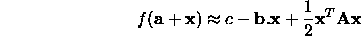The gradient of f near a is given by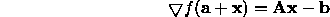If we have just minimized along a direction u then the component of the gradient along u must be zero, thus the gradient itself is perpendicular to u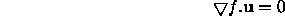As we now move along some direction v the gradient changes by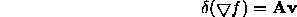In order not to interfere with our u minimization we require that the gradient remain perpendicular to u, ie that the change in gradient itself be perpendicular to u. This is simply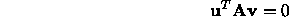Two vectors, u, v, having this property are said to be conjugate. A set of vectors for which this holds for all pairs is a conjugate set.

If we minimize along each of a conjugate set of n directions we will get closer to the minimum efficiently. If the function has an exact quadratic form, one pass through the set will get us exactly to the minimum. Otherwise we must repeat the cycle a number of times.

The problem is, how do we generate such a conjugate set? If f is second-differentiable we can calculate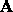and generate the set using algebraic techniques. However, there are much more effective methods available in such cases (see below). If no derivatives are available a method due to Powell can be used which generates a conjugate set a sequence of line minimizations. It starts by assuming a set consisting of the unit directions, then gradually calculates new directions from the results of the search. Each n line minimizations gives an extra conjugate direction, so a full set is derived after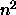line minimizations. Details are given in .

Powell's is the method of choice if we have a reasonable starting approximation, we cannot easily obtain derivatives and the function isn't too noisy.Next: Multi-Dimensional Search (With Up: Multi-Dimensional Search (No Previous: Multi-Dimensional Search (No

Bob Fisher
Fri Mar 28 14:12:50 GMT 1997